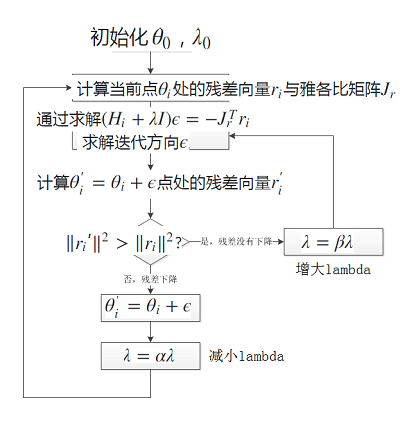# 高斯-牛顿法(Guass-Newton Algorithm)与莱文贝格-马夸特方法(Levenberg–Marquardt algorithm)求解非线性最小二乘问题

$$H = \begin{bmatrix}\frac{\partial^2f}{\partial \theta^2} \end{bmatrix}=\sum\begin{bmatrix}r_i\frac{\partial^2r_i}{\partial\theta^2}+(\frac{\partial r_i}{\partial \theta})(\frac{\partial r_i}{\partial \theta})^T \end{bmatrix}$$观察二阶导数项$$r_i\frac{\partial^2r_i}{\partial\theta^2}$$，因为残差 $$r_i\approx 0$$,因此我们可以认为此项接近于0而舍去。所以Hessian矩阵可以近似写成：$$H\approx\sum\begin{bmatrix}(\frac{\partial r_i}{\partial\theta})(\frac{\partial r_i}{\partial\theta})^T\end{bmatrix}=J_r^TJ_r$$

Levenberg-Marquart 算法

• 当$$\lambda$$增大时，$$H+\lambda I$$趋向于$$\lambda I$$，因此$$\epsilon$$趋向于 $$-\lambda J_r^Tr$$，也就是梯度下降法给出的迭代方向；
• 当$$\lambda$$减小时，$$H+\lambda I$$趋向于$$H$$，$$\epsilon$$趋向于$$-H^{-1}J_r^Tr$$，也就是高斯-牛顿法给出的方向。

$$\lambda$$的大小通过如下规则调节，也就是L-M算法的流程：1. 初始化 $$\theta_0$$，$$\lambda_0$$。
2. 计算当前点$$\theta_i$$处的残差向量$$r_i$$与雅各比矩阵$$J_r$$。
3. 通过求解$$(H_i+\lambda I)\epsilon = -J_r^Tr_i$$求解迭代方向$$\epsilon$$。
4. 计算$$\theta_i^\prime=\theta_i+\epsilon$$点处的残差向量$$r_i^\prime$$。
5. 如果$$\|r_i\prime\|^2>\|r_i\|^2$$?,即残差没有下降，则更新$$\lambda = \beta\lambda$$，增大$$\lambda$$重新回到第三步重新求解新的$$\epsilon$$。如果残差下降，则更新$$\theta_{i+1} = \theta_i+\epsilon$$ ，到第二步，并且降低$$\lambda=\alpha\lambda$$，增大迭代步长。

1.maximum likelihood regression-university of manitoba

2.过拟合与欠拟合-网易公开课：斯坦福大学机器学习课程

3.Using Gradient Descent for Optimization and Learning

4.Numerical Optimization using the Levenberg-Marquardt Algorithm-Los Alamos National Laboratory

5.Circular and Linear Regression Fitting Circles and Lines by Least Squares – Nikolai Chernov – UAB

## 《高斯-牛顿法(Guass-Newton Algorithm)与莱文贝格-马夸特方法(Levenberg–Marquardt algorithm)求解非线性最小二乘问题》上有3条评论

1.Dr.Cup说道：

写的有问题吧？高斯牛顿是泰勒公式的一阶展开，没有汉森矩阵

•whudj说道：

您好，谈一下我的理解，高斯-牛顿法是牛顿法的一种改型，其特点是省略了二阶导数矩阵的计算，采用近似的矩阵取代hessian 矩阵。方法本身还是二阶的。而非对泰勒公式的一阶展开。